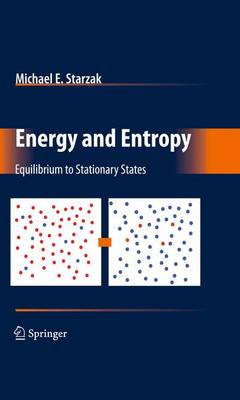•# Energy and Entropy: Equilibrium to Stationary States (Hardback)

(author)
£64.99
Hardback 314 Pages / Published: 06/01/2010
• We can order this

Usually dispatched within 3 weeks

The study of thermodynamics is often limited to classical thermodynamics where minimal laws and concepts lead to a wealth of equations and applications. The resultant equations best describe systems at equilibrium with no temporal or s- tial parameters. The equations do, however, often provide accurate descriptions for systems close to equilibrium. . Statistical thermodynamics produces the same equilibrium information starting with the microscopic properties of the atoms or molecules in the system that correlates with the results from macroscopic classical thermodynamics. Because both these disciplines develop a wealth of information from a few starting postulates, e. g. , the laws of thermodyamics, they are often introduced as independent disciplines. However, the concepts and techniques dev- oped for these disciplines are extremely useful in many other disciplines. This book is intended to provide an introduction to these disciplines while revealing the connections between them. Chemical kinetics uses the statistics and probabilities developed for statistical thermodynamics to explain the evolution of a system to equilibrium. Irreversible thermodynamics, which is developed from the equations of classical thermodyn- ics, centers on distance-dependent forces, and time-dependent ?uxes. The force ?ux equations of irreversible thermodynamics lead are generated from the intensive and extensive variables of classical thermodynamics. These force ?ux equations lead, in turn, to transport equations such as Fick's ?rst law of diffusion and the Nernst Planck equation for electrochemical transport. The book illustrates the concepts using some simple examples.

Publisher: Springer-Verlag New York Inc.
ISBN: 9780387778228
Number of pages: 314
Weight: 1410 g
Dimensions: 235 x 155 x 19 mm
Edition: 2010 ed.

MEDIA REVIEWS

From the reviews:

"Energy and Entropy offers an interesting approach to combining classical and statistical thermodynamics. ... Starzak (chemistry, SUNY Binghamton) frequently mixes statistical examples into the portions of the text that deal with classical thermodynamics. ... The end-of-chapter problems encourage students to apply what they have learned, with worked-out solutions provided at the end of the book. ... Summing Up: Recommended. Upper-division undergraduates through researchers/faculty." (H. Giesche, Choice, Vol. 47 (11), August, 2010)